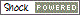四班系统的差异

AAC采购订单差异

(标准的材料费差异)=[sum(发出的材料数量×发出时的标准成本）-sum(发出的材料数量×当前的标准成本）]+[sum(接收的副产品/工具数量×当前的标准成本）-sum(接收的副产品/工具数量×入库时的标准成本）]

(标准的人工费差异)=sum(报告的资源数量×报告时的标准成本）-sum(报告的资源数量×当前的标准成本）

(标准的间接费差异)=[sum(报告的资源数量）×（报告时的标准固定间接费+可变间接费）]-[sum(报告的资源数量）×（当前的标准固定间接费+可变间接费）]

(资源总差异)=sum(报告的资源数量×报告时的标准人工费)+[sum(报告的资源数量)×(报告时标准的固定+可变间接费)]-[(接收的父项数量)×(父项的本层增加标准人工费+固定间接费+可变间接费)]

(累计的副产品/工具成本)=以运行OVAR任务时有效的物料清单为基础的累计成本。

[sum(报告的资源数量-需要的资源数量)*(资源标准的固定间接费+标准的可变间接费)]+[(父项的订货数量-父项的接收数量)*(

(父项本层累计固定间接费+可变间接费)=本层累计固定和可变间接费以运行OVAR任务时的物料清单为基础。

(材料总差异)=[sum(发出的材料数量×发出时的标准成本)-sum(接收的副产品/工具数量×接收时的标准成本)]-(接收的父项数量)×[(父项的标准成本)-(父项的本层标准人工费+固定间接费+可变间接费)]

(订单上的材料子项价值)计算时来自OVAR报告数据=(子项类型的标准累加成本合计=N和子项类型的标准累加成本合计=Z)

(订单上的材料子项价值)计算时来自具有成本数据的单层物料清单(CSLB)屏幕数据=(子项类型的标准累加成本合计=N和子项类型的标准累加成本合计=P)

(材料费累积差异)=[(父项的累积材料费)-((父项标准成本)-(父项本层增加的标准人工费+固定间接费+可变间接费))]*(接收的父项数量)

(父项的累积材料费)=以运行OVAR任务时有效的物料清单为基础的累积材料费。

(人工费累积差异)=[(父项的累积人工费)-(父项本层增加的标准人工费)]*(接收的父项数量)

(父项的累积人工费)=以运行OVAR任务时有效的物料清单为基础的累积人工费。

(间接费累积差异)=[(父项的累积间接费)-(父项本层增加的标准固定间接费+可变间接费)]*(接收的父项数量)

(父项的累积间接费)=以运行OVAR任务时有效的物料清单为基础的累积固定间接费加可变间接费。

[(报告的资源数量-需要的资源数量)*(资源标准人工费)]+[(父项订货数量-父项接收数量)*(父项的本层累积成本)]

(父项的本层累积成本)=以运行OVAR任务时有效的物料清单为基础的本层累积人工费。